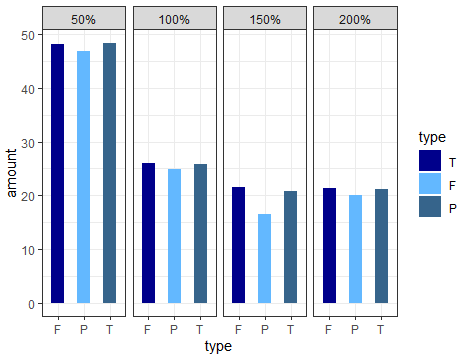4 views

Data:

type    size    amount

T   50%     48.4

F   50%     48.1

P   50%     46.8

T   100%    25.9

F   100%    26.0

P   100%    24.9

T   150%    21.1

F   150%    21.4

P   150%    20.1

T   200%    20.8

F   200%    21.5

P   200%    16.5

I need to plot a bargraph of the above data using ggplot (x-axis -> "type", y-axis -> "amount", group by "size"). When I used the following code, I am not getting the variable "type" and as well as "size" in the order shown in the data. Please see the figure. I have used the following code for that.

ggplot(temp, aes(type, amount , fill=type, group=type, shape=type, facets=size)) +

geom_bar(width=0.5, position = position_dodge(width=0.6)) +

facet_grid(.~size) +

theme_bw() +

scale_fill_manual(values = c("darkblue","steelblue1","steelblue4"),

labels = c("T", "F", "P"))For fixing the order issue, I have used a factor method for the variable "type" using the following. Please see the figure also.

temp\$new = factor(temp\$type, levels=c("T","F","P"), labels=c("T","F","P"))However, now I don't know how to fix the order for the variable "size". It should be 50%, 100%. 150%, and 200%.

by

To fix the order of the facets in your plot, factor the size variable and set the levels as follows:

df\$size_f = factor(df\$size, levels=c('50%','100%','150%','200%'))

For example:

df <- data.frame(type=rep(c("T", "F", "P"), 4),

size=rep(c("50%", "100%", "200%", "150%"), each=3),

amount=c(48.4, 48.1, 46.8,

25.9, 26.0, 24.9,

21.1, 21.4, 20.1,

20.8, 21.5, 16.5))

ggplot(df, aes(type, amount , fill=type, group=type, shape=type, facets=size)) +

geom_bar(width=0.5, position = position_dodge(width=0.6),stat = "identity") +

facet_grid(.~size_f) +

theme_bw() +

scale_fill_manual(values = c("darkblue","steelblue1","steelblue4"),

labels = c("T", "F", "P"))